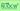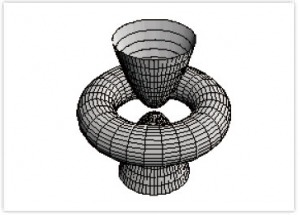# Graphical Information and ComputerLecturer Eisuke KITA, Professor Institute of Liberal Arts & Sciences, 2014 Spring & Fall ( 1st semester ) Students in the school of Informatics and Sciences (Social and Human Science Informatics), Letters, Economics, Education and Law ( 2nd semester ) Students in the school of Informatics and Sciences (Natural Science Informatics), Engineering, Science, Medicine and Agriculture (2・1.5 hours / session 1 session / week 15 weeks / semester)### Course Overview

In the first part of this course, we will be learning about the analytic algorithm of time series data using spreadsheet software. In the second part of this lecture, we will be focusing on the usage of the formula manipulation software, Mathematica. Basic usage for mathematics calculations, creation of graphs and animations, and music composition will be the main topic of our exercise problems.

### Key Features

This lecture is experiential learning. Therefore, Many example problems will be given in the lecture in order to make this lecture interesting. Also example problems will be realistic matters such as earning of department store or stock for your better understanding.

### Course Aims

Main objective of this lecture is to introduce convenient usages of data analysis software and computer computation software. In the first part of the lecture, our main focus will be on the application of spreadsheet software. We will be learning about the analytic method of time series data by using least squares analysis, moving average analysis, correlated analysis and so on. In the second part of the lecture, our main focus will be on Mathematica. We will be learning how to use Mathematica for the linear algebra and calculus calculations. In addition, we will also experience some of the interesting application for making animation and composing music. The contents of the class will be different depending on the students' major and interests.

Mathematics and Information literacy are not required but deeply related to the contents of this course.

### Contents

Lecture will be in the PC lab in the Integrated Research Building. Contents will be as following.

1. Creating Figure and spreadsheet data using Excel
2. Least mean square approximation analysis and moving data average analysis of time series data using Excel
3. Moving data analysis using Excel
4. Correlated analysis using Excel
5. Basics of Mathematica (computational software)
6. Algebra calculations using mathematica
7. Data analysis using Mathematica
8. Creating graphics using Mathematica
9. Creating animation using Mathematica
10. Composing music using Mathematica

### Textbooks

No textbooks are assigned.

### Note

This lecture is experiential learning. Therefore, exercise will be the main part of the lecture and I would like all the students to have fun in the lecture.

### Communication Board:

This course is designed for both beginner and intermediate user of Computer. Therefore this lecture consists of the basics of informatics literacy and further application of computer software on the various analyses. In the first part of the lecture, we will begin with the introduction of e-mail and word processing software. It is because these soft wares will be the most fundamental knowledge for using Computer. Then secondly we will be learning about the method of data analysis using Excel and Mathematica. Since using Mathematica should be the first time for many students, I would like all the students to experience new software and to increase their interests and knowledge thorough experiential learning. Balance of basic materials and intermediate materials varies depending on the needs of the students. If you have any suggestion about the lecture or exercise, it is very welcome.

An Introduction to Programming with Mathematica, Third Edition (Hardcover), Paul R. Wellin, Richard J. Gaylord, Samuel N. Kamin, Cambridge University Press; 3 edition, 2005

Programming in Mathematica, Third Edition (Paperback), Roman Maeder, Addison-Wesley Professional; 3 editions, 1997

### Assignments

Assignments are to solve the example problems given in the lecture.

• Due date of the homework will be one week from the assigned date, or the beginning of the next lecture.
• Tardy paper will be accepted but will get point off for the delay. If the full mark is 1 the maximum score of the tardy report will be 0.5.
• If you do not hand in report you get 0 point for the results of the report.
• If you hand in report whose due date is much earlier, it will not be accepted.

### Course Schedule

Session Contents
1

Guidance

• Setting e-mail environment
• Sending self-introduction for the first report
2

Basics of Excel (creating charts and figures)

• How to make a chart in Excel
• How to make a figure in Excel
3

Least mean square approximation analysis using Excel

• How to apply least mean square approximation analysis for a sample data
4

Correlated analysis using Excel

• How to use correlated analysis for two sets of data
5

Autocorrelation analysis using Excel

• Evaluation of correlation and analysis on periodicity
6

Difference analysis using Excel

• How to analyze time series data
7

Moving Average analysis using Excel

• How to analyze time series data and evaluate the long-term tendency of the data
8

Basics of Mathematica

• What can we do using Mathematica?
9

Algebra calculations using Mathematica

• Why don't we start from familiar example problems in Algebra?
10

Linear Algebra calculations using Mathematica

• Solving familiar linear algebra problems using list function for computing matrix of vector
11

Calculus calculations using Mathematica

• Application of derivation, integrating and solution of differential equations
12

Curve fitting using Mathematica

• Least-squares method and spline function
13

Creating graphics using Mathematica

• Drawing graphics using Mathematica
14

Two dimensional animation using Mathematica

• Parallel displacement and rotational displacement of two-dimensional graphics
15

Composing music using Mathematica

• Lets compose Japanese music?
• In the last part of the lecture, we will compose the famous Japanese music, "Sakura Sakura".

### Lecture Handouts

Note: All files are in Japanese.

Session #2 Basics of Excel

Session #4 Correlated analysis using Excel

Session #5 Autocorrelation analysis using Excel

Session #6 Difference analysis using Excel

Session #7 Moving Average analysis using Excel

Session #8 Basics of Mathematica

Session #9 Algebra calculations using Mathematica

Session #10 Linear Algebra using Mathematica

Session #11 Calculus using Mathematica

Session #12 Curve fitting using Mathematica

Session #13 Graphics using Mathematica

Session #14 Two dimensional animation using Mathematica

Session #15 Composing music using Mathematica